## 15 Questions MCQ Test Mathematical Olympiad Class 8 | Olympiad Test: Data Handling

Description
Attempt Olympiad Test: Data Handling | 15 questions in 30 minutes | Mock test for Class 8 preparation | Free important questions MCQ to study Mathematical Olympiad Class 8 for Class 8 Exam | Download free PDF with solutions
QUESTION: 1

### From a well shuffled deck of deck of 52 cards, one card is drawn at random. What is the probability that the card drawn is a diamond?

Solution:

Total number of all possible outcomes = 52
No. of diamonds = 13
P(getting a diamond) =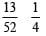QUESTION: 2

### A bag contains 4 red balls, 5 green balls and 7 black balls. They are mixed thoroughly and one ball is drawn at random. What is the probability of getting a black ball?

Solution:

Total number of balls = 4 + 5 + 7 = 16
Number of black balls = 7
P(getting a black ball) = 7/16

QUESTION: 3

### The following data shows the agricultural production in India during a certain year.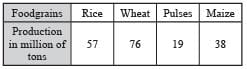Q. What is the central angle for Rice in a pie chart?

Solution:

Total production = 57 + 76 + 19 + 38
=190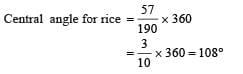QUESTION: 4

The electricity bill in Rupees of 25 houses of a certain locality for a month are given below. 472, 763, 312, 630, 584, 324, 700, 617, 754, 776, 596, 745, 565, 780, 378, 570, 685, 400, 356, 365, 435, 506, 548, 736.

Q. Arrange the data in increasing order and find the frequency of the group 7000-800.

Solution: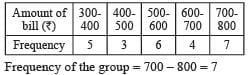QUESTION: 5

The monthly income of a family is ₹ 14, 400 and the central angle for the rent on a pie chart is 100°. What amount shows the rent?

Solution:

Central angle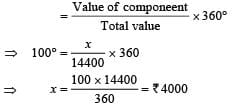QUESTION: 6

In a pie-chart for expenditure in percent incurred in the construction of a house in a city, the central angle for cement is 72°. What is the percentage of cement expenditures ?

Solution: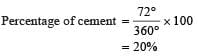QUESTION: 7

In a lottery there are 10 prizes and 20 blanks. A ticket is chosen at random. What is the probability of not getting a prize?

Solution:
QUESTION: 8

From a well shuffled deck of 2 cards, one card is drawn at random. What is probability of getting a red card?

Solution:

Total no. of possible outcomes = 52

Number of red cards = 26.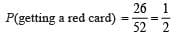QUESTION: 9

In a box of 100 electric bulbs, 8 bulbs are defective. One bulb is taken out at random from the box. What is the probability that the bulb drawn is not defective?

Solution:

Total no. of electric bulbs = 100
No. of defective bulbs = 8
No. of bulbs which are not defective = 100 – 8 = 92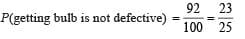QUESTION: 10

One card is drawn at random from a well shuffled deck of 52 cards. What is the probability that the card drawn is a queen?

Solution:

Total no. of possible outcomes = 52
No. of queen = 4.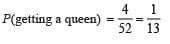QUESTION: 11

A die is thrown. What is the probability of getting 6?

Solution:

Total no. of possible outcomes = 6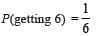QUESTION: 12

From a well shuffled deck of 52 cards. One card is drawn at random. What is the probability of getting a card of black 6?

Solution:

Total no. of possible outcomes = 52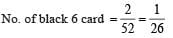QUESTION: 13

The ages of 50 members of the Junior cricket club in a town are as given below. 15, 17, 14, 13, 14, 13, 14, 17, 17, 16, 17, 16, 15, 16, 15, 14, 13, 14, 15, 13, 18, 13, 15, 14, 15, 13, 14, 13, 13, 17, 15, 14, 14, 17, 16, 17, 15, 14, 17, 16, 16, 16, 14, 16, 13, 18, 16, 15, 14, 14.

Q. What percentage of members is in the 15-16 age group?

Solution: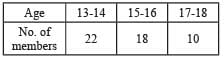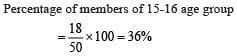QUESTION: 14

The pie-chart represents the amount spent on different sports by a sport club in a year. If the total money spent by the club is ₹ 10,800. Find the amount spent on cricket.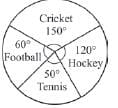Solution: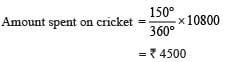QUESTION: 15

Mohan spends 40% of his monthly income on food items, 20% on house rent and 30% on miscellaneous items. He saves 10% of his income every month. What is the central angle for house rent on pie-chart?

Solution: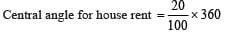Use Code STAYHOME200 and get INR 200 additional OFF Use Coupon Code